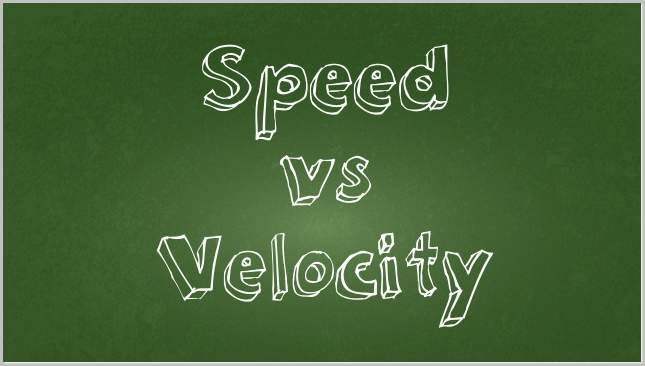# Difference between Speed and Velocity

Speed and velocity are often used interchangeably in common conversation. However, in the physical sciences (and especially in physics), the two terms actually refer to specific and different forms of measurement. In particular, speed refers to the rate that an object changes its position over time; that is, the distance it travels divided by the time it has been travelling. Velocity, however, refers to the rate of change in a particular direction, or, in the parlance of physics, along a particular vector.

Put simply, to say that an object is travelling at 10 miles per hour is to refer to its speed, whereas to say that the same object is travelling at 10 miles per hour due northwest is to add the vector of travel, and therefore to refer to velocity. In general, basic physics will discuss velocity more often than speed. Velocity is also calculated based on the change in position (i.e. the distance travelled) over time; acceleration can then be calculated by dividing a change in velocity over the time of the change.

## Speed vs. VelocitySPEED

Speed is a scalar measurement of distance travelled over time – that is, a measure of the change in position regardless of a specific change in the coordinates of the object. In imperial systems of measurement, as in the United States, speed is measured in terms of feet per second and miles per hour. However, in metric regions, it is measured in terms of metres per second and kilometres (1000 metres) per hour.

According to our best understanding of physics today, objects can travel at speeds up to just under the speed of light, which is 670 million miles or 1080 million kilometres per hour. An object travelling at the speed of light would, at least theoretically, require infinite energy, and therefore infinite mass.

VELOCITY

Whereas speed is a scalar measurement, physics is a vector measurement: it considers not only speed but also the direction in which the object is travelling. Provided that this direction is incorporated, however, the units of measurement are equivalent: that is, speed, like velocity, is measured in terms of feet and metres per second, and miles and kilometres per hour.

Velocity allows for more complicated measurements than speed, including the ultimate velocity of an object being pushed by forces from multiple vectors. For example, an aircraft which is exerting a strong force due north due to its engines, but is also being pushed by a brisk wind due west, will actually travel at a combined velocity, in a direction somewhere slightly to the west of due north (i.e. north-northwest).